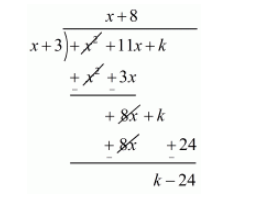# For what value of k, is −3 a zero of the polynomial x2 + 11x + k?`
Question:

For what value of $k$, is $-3$ a zero of the polynomial $x^{2}+11 x+k$ ?

Solution:

We know that if $x=\alpha$ is zeros polynomial, then $x-\alpha$ is a factor of $f(x)$

Since $-3$ is zero of $f(x)$. Therefore $x+3$ is a factor of $f(x)$

Now, we divide $f(x)=x^{2}+11 x+k$ by $g(x)=x+3$ to find the value of $k$.Now, Remainder $=0$

$k-24=0$

$k=24$

Hence, the value of $k$ is 24 .